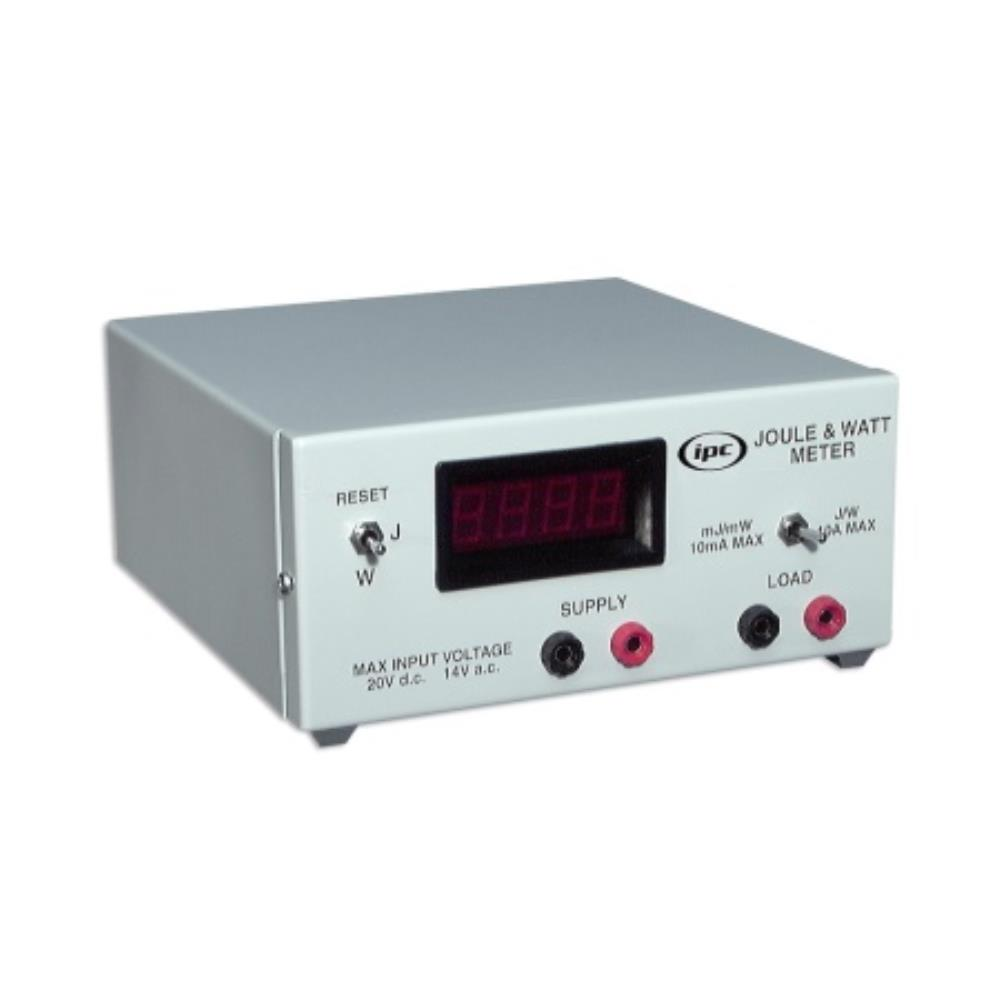# IPC Joule + Watt Meter

Code: EL10409 N1-1

Capable of making direct measurement of electrical energy or power supplied to a load and is therefore a valuable tool for pupil based work as well as simplifying many demonstrations. The direct re...

£192.43 EACH
List PriceCapable of making direct measurement of electrical energy or power supplied to a load and is therefore a valuable tool for pupil based work as well as simplifying many demonstrations. The direct readings mean that the need for voltmeter, ammeter, stop watches and calculators is eliminated and fast and accurate results can be obtained. The science understanding can therefore be gained from scientific investigations rather than mathematical manipulation, making it ideal for pupil based investigations at GCSE, Standard Grade level and A-level in science and technology courses. Its many uses include experiments with an electrical heater, determination of energy stored in a capacitor, calculating the power supplied by a DC source to a resistive load and measurement of the specific latent heat of steam.

• Capable of measuring energies from 1mJ to 9999J or power from 1mW to 200W in AC or DC circuits
• Two ranges selected by a switch labelled mJ/mW and J/W are available
• Auto-ranging four digit display will give direct readings of energy or power
• Maximum current input is 10A
• All electrical connections are via 4mm sockets

 Electrical Supply 220-240VAC, 50-60Hz Dimensions 179 x 190 x 85mm Weight 1.6kg Max. Input Voltage 20VDC, 14VAC (rms) Input Resistance 2MΩ (no load) Max. Load Currents J/W range = 10A, mJ/mW range = 10mA Max. Energies J range = 9999J, mJ range = 9999mJ Max. Powers W range = 200W, mW range = 200mW Accuracy Typically ±5% of full scale reading